# 前言

1. 熟悉图像处理常用的卷积
2. 熟悉高斯模糊、Sobel算子

DirectX11 With Windows SDK完整目录

Github项目源码

# 图像卷积

$H_{ij}=\sum_{r=-a}^{a}\sum_{c=-b}^{b}W_{rc}P_{i-r,j-c}$

$H_{ij}=\sum_{r=-a}^{a}\sum_{c=-b}^{b}W_{rc}P_{i+r,j+c}$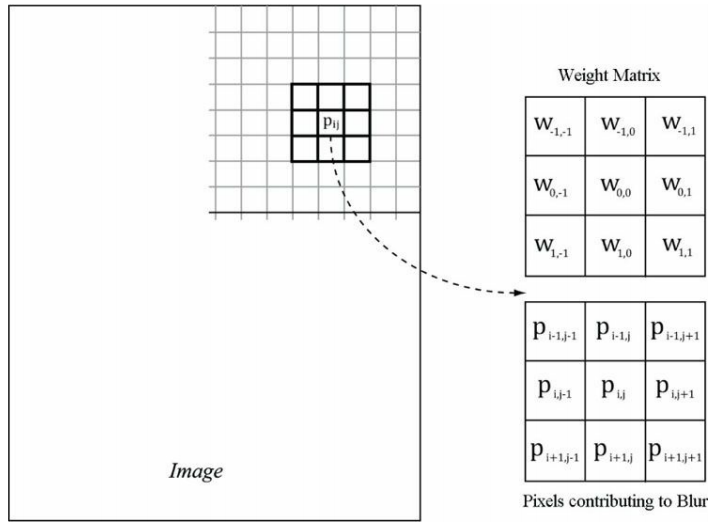# 图像模糊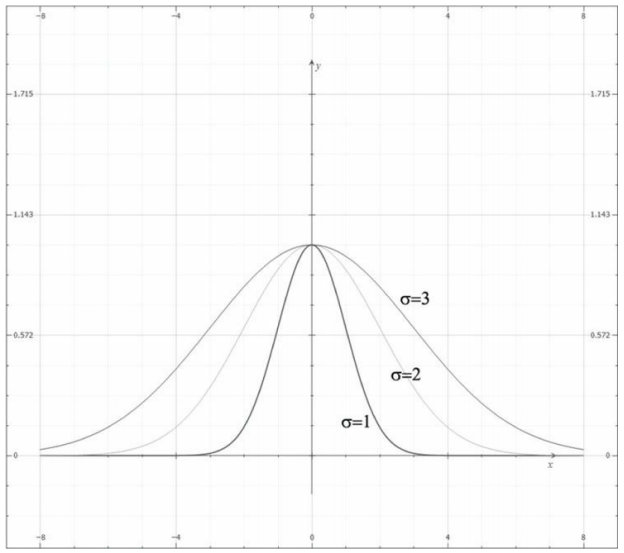$G(x)=exp(-\frac{x^2}{2\sigma^2})=e^{-\frac{x^2}{2\sigma^2}}$

\begin{align}G(-2)&=exp(-\frac{(-2)^2}{2})=e^{-2} \\G(-1)&=exp(-\frac{(-1)^2}{2})=e^{-\frac{1}{2}} \\G(0)&=exp(0)=1 \\G(1)&=exp(-\frac{1^2}{2})=e^{-\frac{1}{2}} \\G(2)&=exp(-\frac{2^2}{2})=e^{-2}\end{align}

\begin{align} \sum_{x=-2}^{x=2}G(x)&=G(-2)+G(-1)+G(0)+G(1)+G(2)\\ &=1+2e^{-\frac{1}{2}}+2e^{-2}\\ &\approx 2.48373 \end{align}

\begin{align} w_{-2}&=\frac{G(-2)}{\sum_{x=-2}^{x=2}G(x)}\approx 0.0545\\ w_{-1}&=\frac{G(-1)}{\sum_{x=-2}^{x=2}G(x)}\approx 0.2442\\ w_{0}&=\frac{G(0)}{\sum_{x=-2}^{x=2}G(x)}\approx 0.4026\\ w_{1}&=\frac{G(1)}{\sum_{x=-2}^{x=2}G(x)}\approx 0.2442\\ w_{2}&=\frac{G(2)}{\sum_{x=-2}^{x=2}G(x)}\approx 0.0545\\ \end{align}

\begin{align} G(x, y) &= G(x)\cdot G(y) \\ &=exp(-\frac{x^2}{2\sigma^2})\cdot exp(-\frac{y^2}{2\sigma^2}) \\ &=e^{-\frac{x^2+y^2}{2\sigma^2}} \end{align}

$\begin{bmatrix} G(-1)G(-1) & G(-1)G(0) & G(-1)G(1) \\ G(0)G(-1) & G(0)G(0) & G(0)G(1) \\ G(1)G(-1) & G(1)G(0) & G(1)G(1) \\ \end{bmatrix} = \begin{bmatrix} G(-1) \\ G(0) \\ G(1) \\ \end{bmatrix}\begin{bmatrix} G(-1) & G(0) & G(1) \\ \end{bmatrix}$

1. 通过1D横向模糊将输入的图像I进行模糊处理：$I_H=Blur_H(I)$
2. 对上一步输出的结果再次进行1D纵向模糊处理：$Blur(I)=Blur_V(I_H)$

$Blur(I)=Blur_V(Blur_H(I))$

## 实现原理

1. 给纹理A绑定SRV作为输入，并且给纹理T0绑定UAV作为输出。
2. 调度线程组进行横向模糊操作。完成后，纹理T0存储了横向模糊的结果
3. 解绑纹理T0的UAV，将它的SRV作为输入。
4. 若用户指定了UAV，并且模糊次数为1，则将该UAV作为输出；否则由于后续还需要进行混合，则将纹理T1的UAV作为输出。
5. 调度线程组进行纵向模糊操作。若当前为最后一次模糊，且用户指定了UAV，则该UAV的纹理将保存最终的结果；否则T1保存了当前模糊的结果。解绑UAV后，若仍有剩余模糊次数，则将纹理T1绑定SRV作为输入，并给纹理T0绑定UAV作为输出，回到步骤2继续；否则就再解绑SRV后结束。

1D纵向模糊于上述1D横向模糊的情况相似。在纵向模糊过程中，线程组就像由256个线程构成的垂直线段，每个线程只负责图像中一个像素的模糊运算。因此，为了使图像中的每个像素都能得到模糊处理，我们需要在y方向上调度$ceil(\frac{h}{256})$个线程组，并在x方向上调度w个线程组。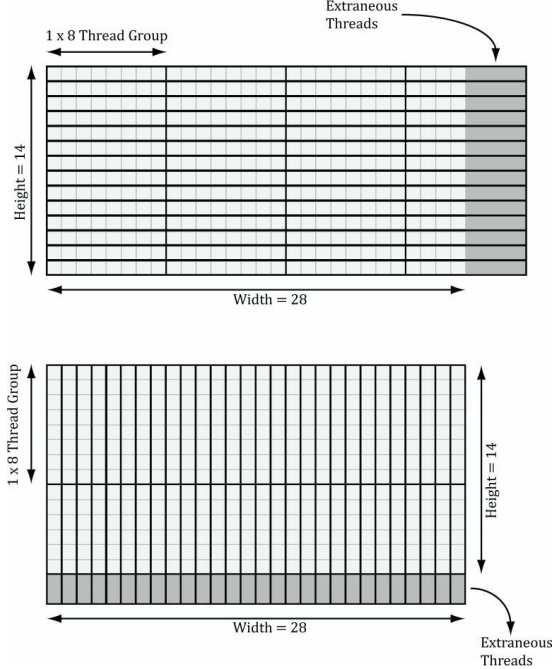BlurFilter::Execute不仅计算出了每个方向要调度的线程组数量，还开启了计算着色器的模糊运算：

void BlurFilter::Execute(ID3D11DeviceContext* deviceContext, ID3D11ShaderResourceView* inputTex, ID3D11UnorderedAccessView* outputTex, UINT blurTimes)
{
if (!deviceContext || !inputTex || !blurTimes)
return;

// 设置常量缓冲区
D3D11_MAPPED_SUBRESOURCE mappedData;
memcpy_s(mappedData.pData, sizeof m_CBSettings, &m_CBSettings, sizeof m_CBSettings);
deviceContext->Unmap(m_pConstantBuffer.Get(), 0);

ID3D11UnorderedAccessView* nullUAV = { nullptr };
ID3D11ShaderResourceView* nullSRV = { nullptr };
// 第一次模糊
// 横向模糊

deviceContext->Dispatch((UINT)ceilf(m_Width / 256.0f), m_Height, 1);
deviceContext->CSSetUnorderedAccessViews(0, 1, nullUAV, nullptr);
// 纵向模糊
if (blurTimes == 1 && outputTex)
deviceContext->CSSetUnorderedAccessViews(0, 1, &outputTex, nullptr);
else
deviceContext->Dispatch(m_Width, (UINT)ceilf(m_Height / 256.0f), 1);
deviceContext->CSSetUnorderedAccessViews(0, 1, nullUAV, nullptr);

// 剩余模糊次数
while (--blurTimes)
{
// 横向模糊

deviceContext->Dispatch((UINT)ceilf(m_Width / 256.0f), m_Height, 1);
deviceContext->CSSetUnorderedAccessViews(0, 1, nullUAV, nullptr);
// 纵向模糊
if (blurTimes == 1 && outputTex)
deviceContext->CSSetUnorderedAccessViews(0, 1, &outputTex, nullptr);
else
deviceContext->Dispatch(m_Width, (UINT)ceilf(m_Height / 256.0f), 1);

deviceContext->CSSetUnorderedAccessViews(0, 1, nullUAV, nullptr);
}
// 解除剩余绑定

}


## HLSL代码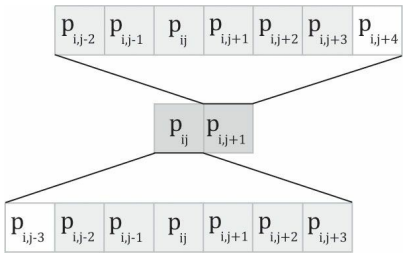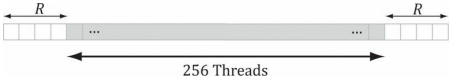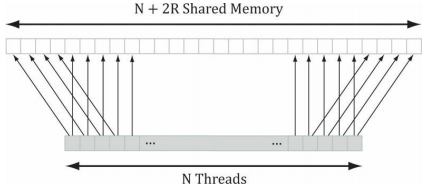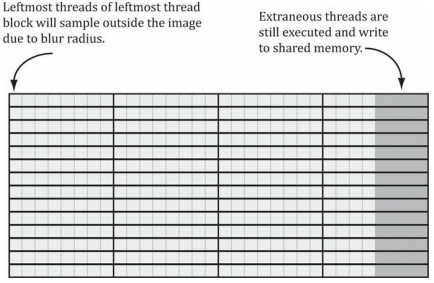// Blur.hlsli
cbuffer CBSettings : register(b0)
{

// 最多支持19个模糊权值
float w0;
float w1;
float w2;
float w3;
float w4;
float w5;
float w6;
float w7;
float w8;
float w9;
float w10;
float w11;
float w12;
float w13;
float w14;
float w15;
float w16;
float w17;
float w18;
}

Texture2D g_Input : register(t0);
RWTexture2D<float4> g_Output : register(u0);

static const int g_MaxBlurRadius = 9;

#define N 256
#define CacheSize (N + 2 * g_MaxBlurRadius)

// Blur_Horz_CS.hlsl
#include "Blur.hlsli"

groupshared float4 g_Cache[CacheSize];

{
// 放在数组中以便于索引
float g_Weights =
{
w0, w1, w2, w3, w4, w5, w6, w7, w8, w9,
w10, w11, w12, w13, w14, w15, w16, w17, w18
};

// 通过填写本地线程存储区来减少带宽的负载。若要对N个像素进行模糊处理，根据模糊半径，
// 我们需要加载N + 2 * BlurRadius个像素

// 线程都多采集一个像素数据
{
// 对于图像左侧边界存在越界采样的情况进行钳位(Clamp)操作
int x = max(DTid.x - g_BlurRadius, 0);
g_Cache[GTid.x] = g_Input[int2(x, DTid.y)];
}

if (GTid.x >= N - g_BlurRadius)
{
// 对于图像左侧边界存在越界采样的情况进行钳位(Clamp)操作
// 震惊的是Texture2D居然能通过属性Length访问宽高
int x = min(DTid.x + g_BlurRadius, g_Input.Length.x - 1);
g_Cache[GTid.x + 2 * g_BlurRadius] = g_Input[int2(x, DTid.y)];
}

// 将数据写入Cache的对应位置
// 针对图形边界处的越界采样情况进行钳位处理
g_Cache[GTid.x + g_BlurRadius] = g_Input[min(DTid.xy, g_Input.Length.xy - 1)];

// 等待所有线程完成任务
GroupMemoryBarrierWithGroupSync();

// 开始对每个像素进行混合
float4 blurColor = float4(0.0f, 0.0f, 0.0f, 0.0f);
{
int k = GTid.x + g_BlurRadius + i;

blurColor += g_Weights[i + g_BlurRadius] * g_Cache[k];
}

g_Output[DTid.xy] = blurColor;
}


Blur_Vert_CS.hlsl与上面的代码类似，就不再放出。

# 索贝尔算子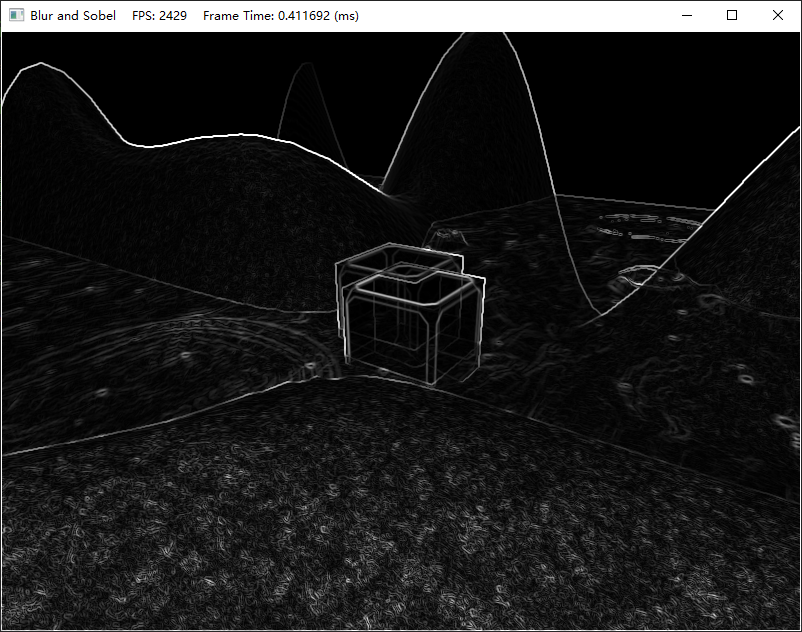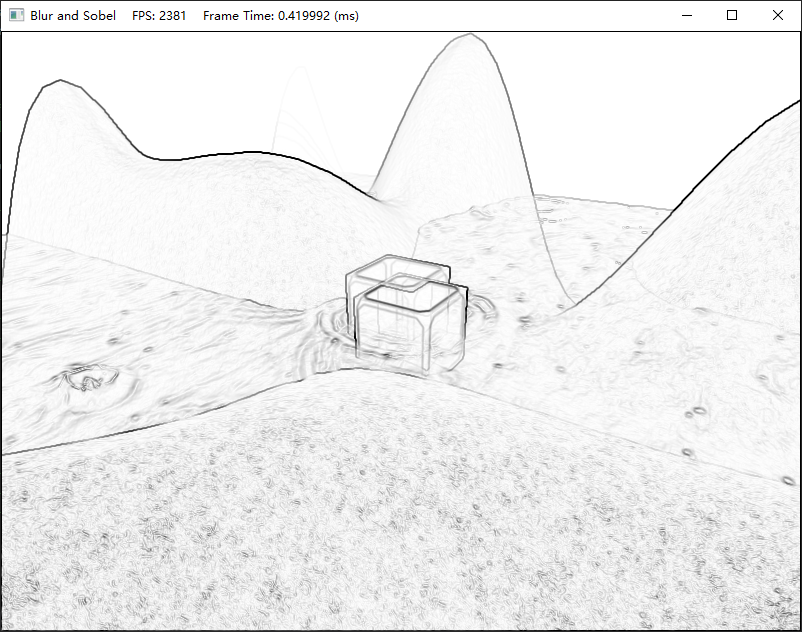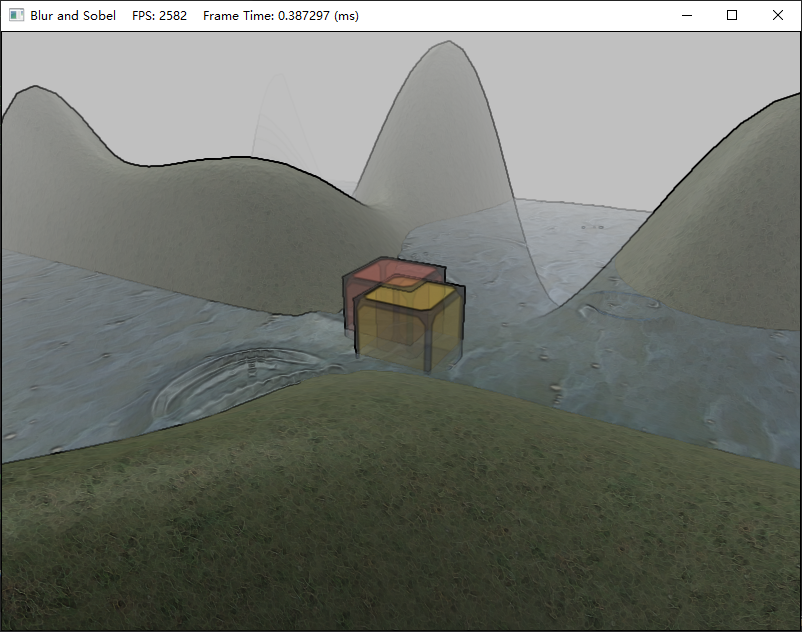$G_x = \Delta_x f(x, y) = [f(x-1,y+1)+2f(x,y+1)+f(x+1,y+1)]-[f(x-1,y-1)+2f(x,y-1)+f(x+1,y-1)] \\ G_y = \Delta_y f(x, y) = [f(x-1,y-1)+2f(x-1,y)+f(x-1,y+1)]-[f(x+1,y-1)+2f(x+1,y)+f(x+1,y+1)]$

## HLSL代码

// Sobel_CS.hlsl
Texture2D g_Input : register(t0);
RWTexture2D<float4> g_Output : register(u0);

// 将RGB色转化为灰色
float3 RGB2Gray(float3 color)
{
return (float3) dot(color, float3(0.299f, 0.587f, 0.114f));
}

{
// 采集当前待处理像素及相邻的八个像素
float4 colors;
for (int i = 0; i < 3; ++i)
{
for (int j = 0; j < 3; ++j)
{
int2 xy = DTid.xy + int2(-1 + j, -1 + i);
colors[i][j] = g_Input[xy];
}
}

// 针对每个颜色通道，利用索贝尔算子估算出关于x的偏导数近似值
float4 Gx = -1.0f * colors - 2.0f * colors - 1.0f * colors +
1.0f * colors + 2.0f * colors + 1.0f * colors;

// 针对每个颜色通道，利用索贝尔算子估算出关于y的偏导数的近似值
float4 Gy = -1.0f * colors - 2.0f * colors - 1.0f * colors +
1.0f * colors + 2.0f * colors + 1.0f * colors;

// 梯度向量即为(Gx, Gy)。针对每个颜色通道，计算出梯度大小（即梯度的模拟）
// 以找到最大的变化率
float4 mag = sqrt(Gx * Gx + Gy * Gy);

// 将梯度陡峭的边缘处绘制为黑色，梯度平坦的非边缘处绘制为白色
mag = 1.0f - float4(saturate(RGB2Gray(mag.xyz)), 0.0f);

g_Output[DTid.xy] = mag;

}


// VS使用Basic_VS_2D
// Composite_PS.hlsl
Texture2D g_BaseMap : register(t0); // 原纹理
Texture2D g_EdgeMap : register(t1); // 边缘纹理
SamplerState g_SamLinearWrap : register(s0); // 线性过滤+Wrap采样器
SamplerState g_SamPointClamp : register(s1); // 点过滤+Clamp采样器

float4 PS(float4 posH : SV_Position, float2 tex : TEXCOORD) : SV_Target
{
float4 c = g_BaseMap.SampleLevel(g_SamPointClamp, tex, 0.0f);
float4 e = g_EdgeMap.SampleLevel(g_SamPointClamp, tex, 0.0f);
// 将原始图片与边缘图相乘
return c * e;
}



# 演示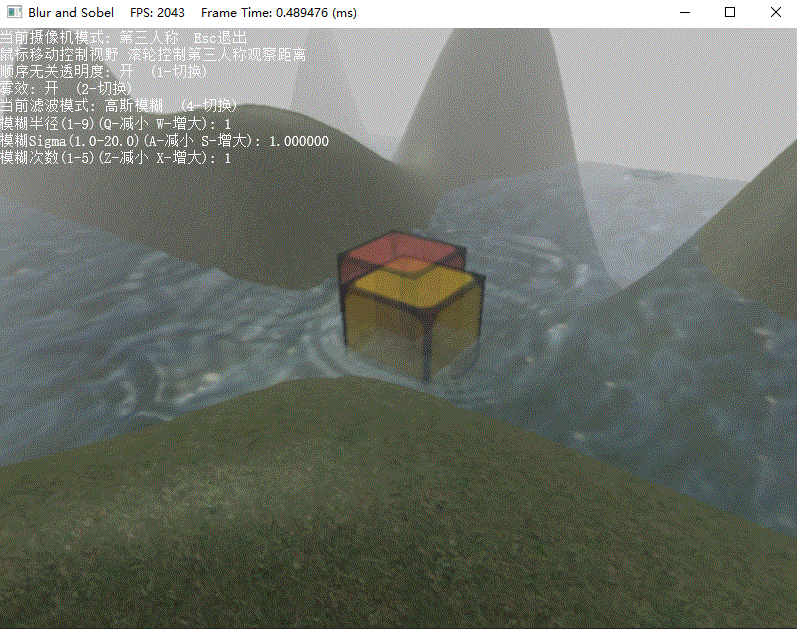# 调试问题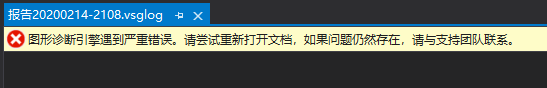# 练习题

1. 对图像进行模糊处理是一种昂贵的操作，它所花费的时间于待处理的图像大小息息相关。一般情况下，在把场景渲染到离屏纹理的时候，我们通常会将离屏纹理的大小设为后备缓冲区尺寸的1/4.也就是说，假如后备缓冲区的大小为800x600，则离屏纹理的尺寸将为400x300.这样一来不仅能加快离屏纹理的绘制速度（即减少了需要填充的像素数量），而且能同时提升模糊图像的处理速度（需要模糊的像素也就更少）。另外，当纹理从1/4的屏幕分辨率拉伸为完整大屏幕分辨率时，纹理放大过滤器也会执行一些额外的模糊操作。

现在尝试修改项目，让BlurFilter的分辨率为400x300，实现上述内容。

提示：TextureRender开启mipmaps，并将mip等级为1的纹理子资源作为SRV。

2. 尝试添加Composite_VS.hlsl，将绘制整个屏幕的6个顶点直接放在顶点着色器中，然后只使用SV_VertexID作为顶点着色器的形参来绘制。

3. 研究双边模糊（双边滤波器，bilateral blur）计数，并用计算着色器加以实现。

DirectX11 With Windows SDK完整目录

Github项目源码

posted @ 2020-02-14 21:46  X_Jun  阅读(1068)  评论(0编辑  收藏  举报
levels of contents